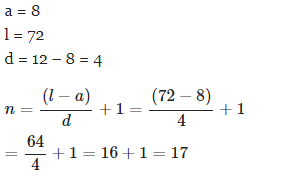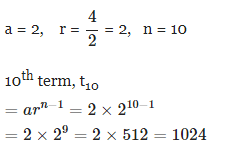# Basic Concepts of Sequence and SeriesLast Updated on Nov 3, 2020

### Sequence

A sequence is a set of values which are in a particular order. In simple words, we can say that a succession of numbers formed according to some definite rule is called a sequence.

Mathematically, a sequence is defined as a map whose domain is the set of natural numbers (which may be finite or infinite) and the range may be the set of real numbers or complex numbers. We can represent it as

f: N → X, where N is the set of naturals. The sequence is finite or infinite depending on whether the set ‘N’ is finite or infinite. If ‘X’ is the set of real numbers then f is said to be a real valued sequence while if the set X is of complex numbers, then f is termed as a sequence of complex numbers.

A set of numbers arranged in a definite order according to some definite rule is called a sequence or a sequence is a function whose domain is the set N of natural numbers. It is customary to denote a sequence by a letter a and the image a(nor t(n). Indicated sum of the terms in a sequence is called a series. The result of performing the additions is the sum of the series. The indicated sum a1 + a2 + a3 + ..... +a1 + a2 + a3 + ….. + an of the terms of a sequence. Here, a1, a2, a3, ...., an is called a series.

#### Sequence and Pattern

If the terms of a sequence follow a particular pattern or a rule, then such a sequence is termed as a progression. Basically, there are three types of progressions:

(i) Arithmetic Progression (A.P.)
(ii) Geometric Progression (G.P.)
(iii) Harmonic Progression (H.P.)

The questions in exams mainly are focused on AP and GP.

#### Arithmetic Progression(AP)

Arithmetic progression(AP) or arithmetic sequence is a sequence of numbers in which each term after the first is obtained by adding a constant, d to the preceding term. The constant d is called common difference.

An arithmetic progression is given by a, (a + d), (a + 2d), (a + 3d), …
where a = the first term , d = the common difference
If a, b, c are in AP, 2b = a + c

tn = a + (n – 1)d
where tn = nth term, a= the first term , d= common difference

Example:
Find the number of terms in the series 8, 12, 16, . . .72#### Geometric progression(GP)

Geometric Progression(GP) or Geometric Sequence is sequence of non-zero numbers in which the ratio of any term and its preceding term is always constant.

A geometric progression(GP) is given by a, ar, ar2, ar3, …
where a = the first term , r = the common ratio
If a, b, c are in GP, b2 = ac

where tn = nth term, a= the first term , r = common ratio, n = number of terms

Example: Find the 10th term in the series 2, 4, 8, 16, …### Summary

In this post, we have explained basic concepts of sequence and series with detailed examples.

If you liked this post, let us know in the comment section below.